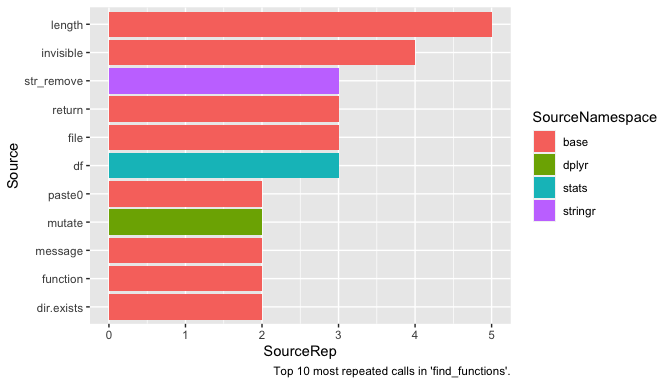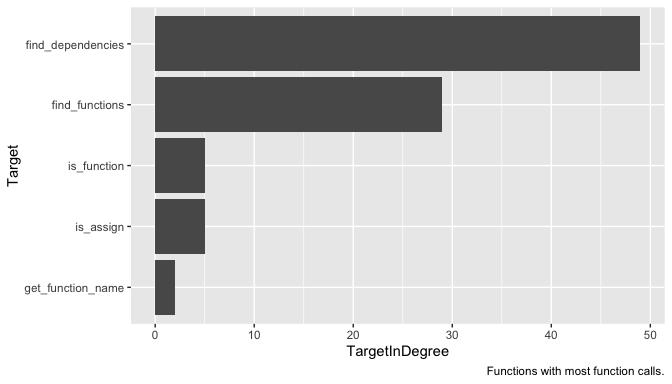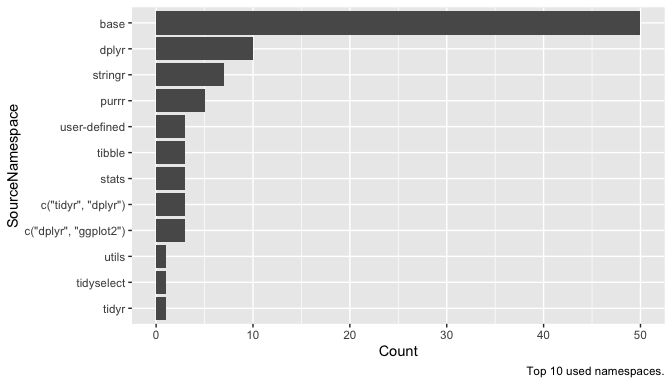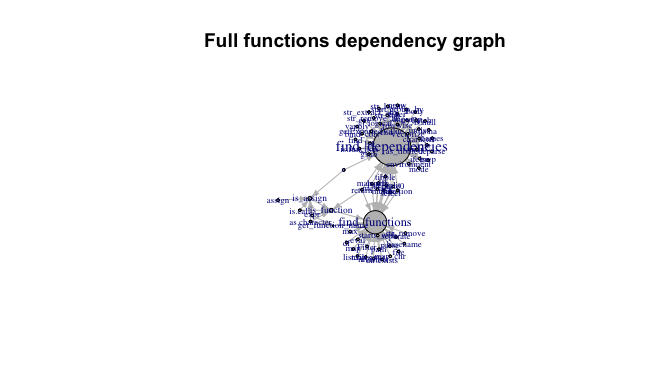# functiondependsThe goal of functiondepends is to allow for tidy exploration of unstructured codebase without evaluation of code.

## Installation

One can install `functiondepends` from CRAN:

``install.packages("functiondepends")``

or development version from GitHub:

``````# install.packages("devtools")
devtools::install_github("jakubsob/functiondepends")``````

## Examples

``````library(functiondepends)
# Create environment for loaded functions
envir <- new.env()
# Search recursively current directory
functions <- find_functions(".", envir = envir, recursive = TRUE)``````
``````functions
#> # A tibble: 5 × 3
#>   Path  Function          SourceFile
#>   <chr> <chr>             <chr>
#> 1 R     find_dependencies find-dependencies.R
#> 2 R     is_function       find-functions.R
#> 3 R     get_function_name find-functions.R
#> 4 R     is_assign         find-functions.R
#> 5 R     find_functions    find-functions.R``````

Search for dependencies of function `find_functions` within parsed functions:

``````dependency <- find_dependencies("find_functions", envir = envir, in_envir = TRUE)
dependency
#> # A tibble: 2 × 5
#>   Source            SourceRep SourceNamespace Target         TargetInDegree
#>   <chr>                 <int> <chr>           <chr>                   <int>
#> 1 get_function_name         1 user-defined    find_functions              2
#> 2 is_function               1 user-defined    find_functions              2``````

Note that `SourceNamespace` column has value `user-defined` as the functions are searched within source of the package.

Search for all dependencies of `find_functions` function:

``````library(ggplot2)
library(dplyr)

dependency <- find_dependencies("find_functions", envir = envir, in_envir = FALSE)
dependency %>%
slice_max(SourceRep, n = 10) %>%
mutate(Source = reorder(Source, SourceRep)) %>%
ggplot(aes(x = Source, y = SourceRep, fill = SourceNamespace)) +
geom_col() +
coord_flip() +
labs(caption = "Top 10 most repeated calls in 'find_functions'.")``````Note that name `df` is often used to store object of type `data.frame`. `df` is also a name of F distribution density function from `stats` package. If you suspect that given function ought not to use a specific package, see the source code of function to check the context. To do so, one can execute `find_dependencies` function with `add_info` argument set to `TRUE`.

``````library(tidyr)

dependency <- find_dependencies("find_functions", envir = envir, in_envir = FALSE, add_info = TRUE)
dependency %>%
filter(SourceNamespace == "stats") %>%
select(Source, SourcePosition, SourceContext) %>%
unnest(c(SourcePosition, SourceContext))
#> # A tibble: 6 × 3
#>   Source SourcePosition SourceContext
#>   <chr>           <dbl> <chr>
#> 1 df                 10 "    df <- purrr::map_dfr(sourceFiles, function(file) {"
#> 2 df                 19 "    source_name <- basename(df\$Path)"
#> 3 df                 21 "    df <- df %>% dplyr::mutate(Path = stringr::str_rem…
#> 4 df                 23 "        paths <- stringr::str_split(df\$Path, \"/|\\\\\…
#> 5 df                 25 "        df <- tidyr::separate(df, \"Path\", into = pas…
#> 6 df                 27 "    df %>% dplyr::mutate(SourceFile = source_name)"``````

One can see that indeed `df` is not a call to function `stats::df`.

``````dependency <- find_dependencies(unique(functions\$Function), envir = envir, in_envir = FALSE)
dependency %>%
distinct(Target, TargetInDegree) %>%
mutate(Target = reorder(Target, TargetInDegree)) %>%
ggplot(aes(x = Target, y = TargetInDegree)) +
geom_col() +
coord_flip() +
labs(caption = "Functions with most function calls.")````````````dependency <- find_dependencies(unique(functions\$Function), envir = envir, in_envir = FALSE)
dependency %>%
group_by(SourceNamespace) %>%
tally(name = "Count") %>%
slice_max(Count, n = 10) %>%
mutate(SourceNamespace = reorder(SourceNamespace, Count)) %>%
ggplot(aes(x = SourceNamespace, y = Count)) +
geom_col() +
coord_flip() +
labs(caption = "Top 10 used namespaces.")``````See which user-defined functions depend most on other user-defined functions within searched codebase.

``````dependency <- find_dependencies(unique(functions\$Function), envir = envir, in_envir = TRUE)
dependency %>%
distinct(Target, TargetInDegree) %>%
arrange(-TargetInDegree)
#> # A tibble: 5 × 2
#>   Target            TargetInDegree
#>   <chr>                      <dbl>
#> 1 find_functions                 2
#> 2 is_function                    1
#> 3 find_dependencies              0
#> 4 get_function_name              0
#> 5 is_assign                      0``````
``````library(igraph)

edges <- dependency %>%
select(Source, Target) %>%
na.omit()

vertices <- unique(c(dependency\$Source, dependency\$Target))
vertices <- vertices[!is.na(vertices)]

g <- graph_from_data_frame(d = edges, vertices = vertices)
deg <- degree(g, mode = "in")
V(g)\$size <- deg * 10 + 5
V(g)\$label.cex <- (degree(g, mode = "in", normalized = TRUE) + 1)

plot(
g,
vertex.color = "grey",
edge.color = "grey",
edge.arrow.size = .4,
main = "Functions dependency graph"
)````````````dependency <- find_dependencies(unique(functions\$Function), envir = envir, in_envir = FALSE)
edges <- dependency %>%
select(Source, Target) %>%
na.omit()
vertices <- unique(c(edges\$Source, edges\$Target))

g <- graph_from_data_frame(edges)
deg <- degree(g, mode = "in")
V(g)\$size <- deg
V(g)\$label.cex <- (degree(g, mode = "in", normalized = TRUE) + 1) / 1.8

plot(
g,
vertex.color = "grey",
edge.color = "grey",
edge.arrow.size = .4,
main = "Full functions dependency graph"
)``````